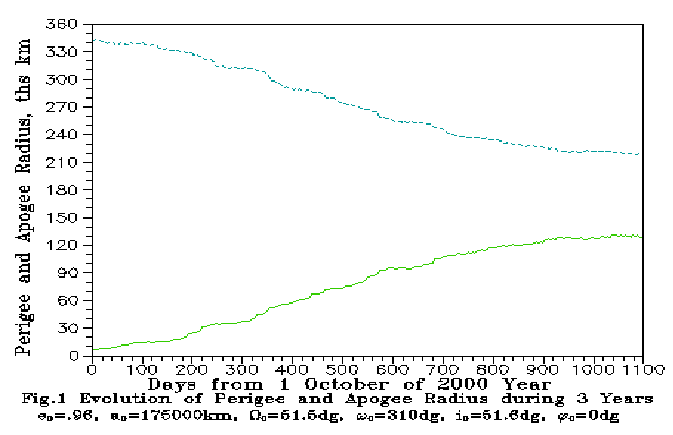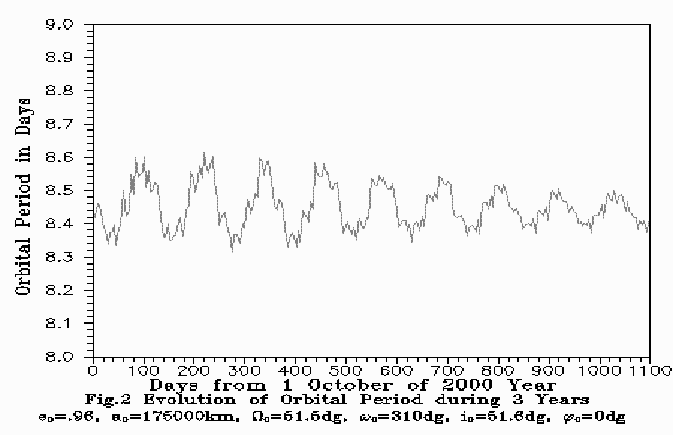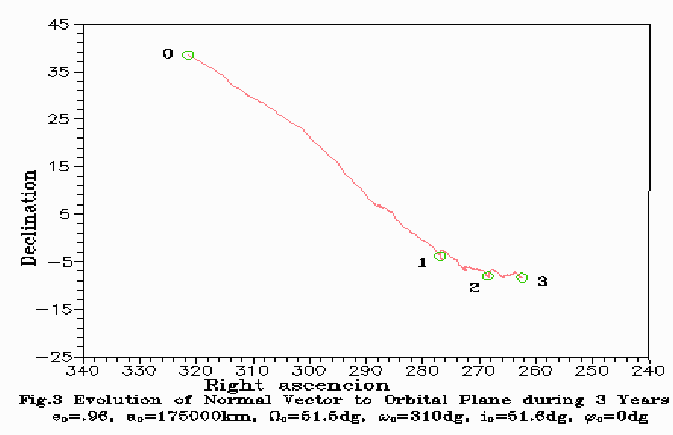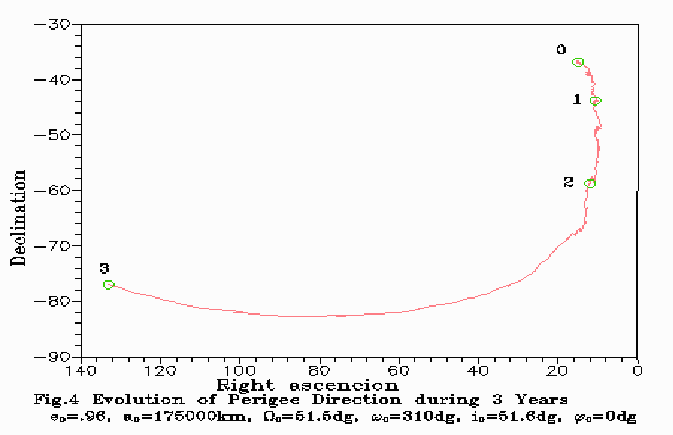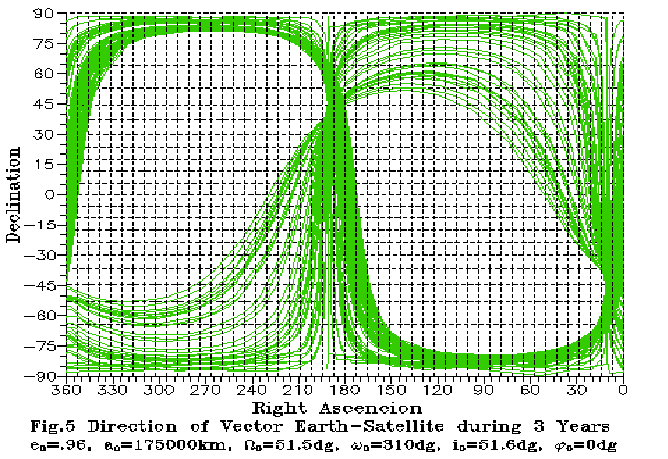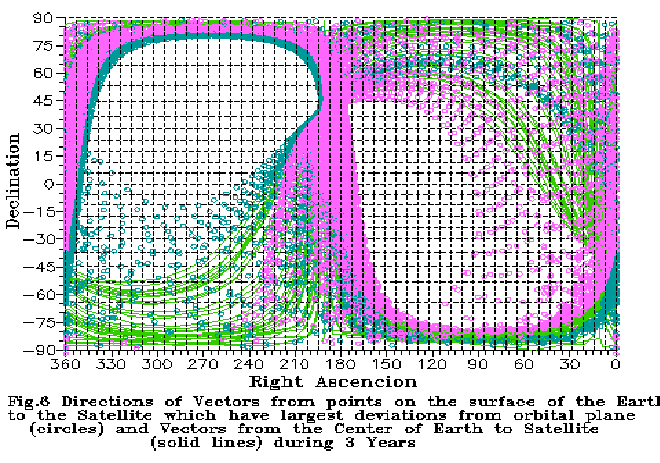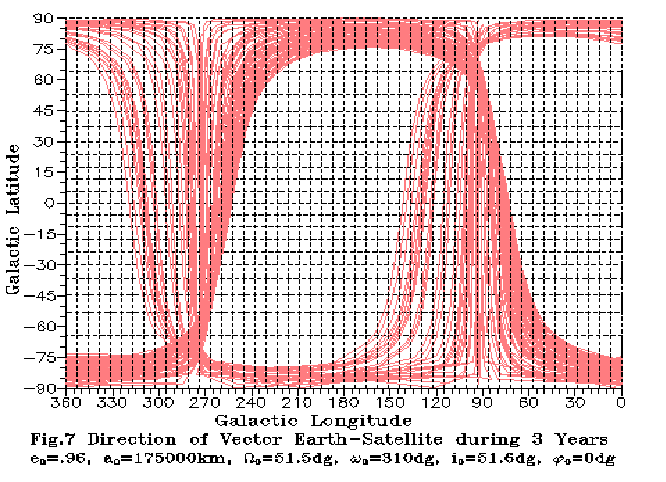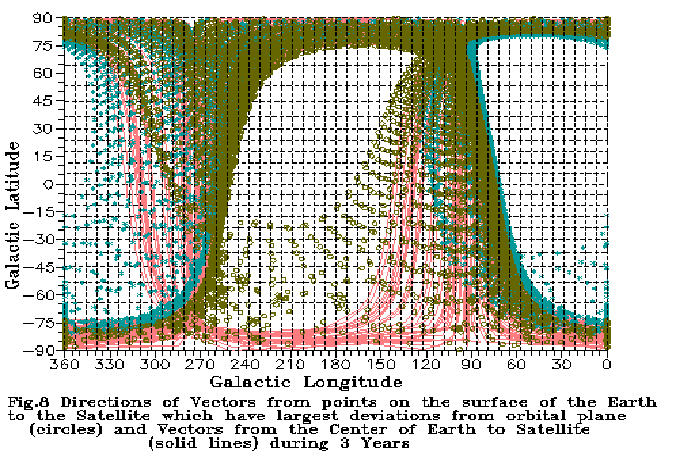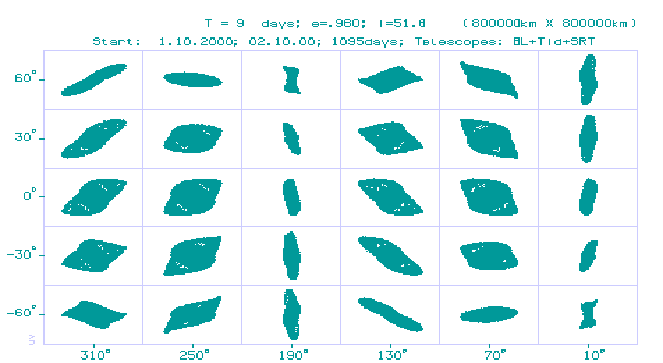MEMORANDUM

Parameters of the Moon-perturbed orbit

Astro Space Center

of the P.N. Lebedev Physical Institute, Moscow, Russia,

March 1998

The launch scheme is adapted to the power capability of the rocket launcher Proton and injection stage. The perigee altitude > 500 km was taken as a limitation. From orbits consistent with this limitation and with the date of the launch October 1, 2000 as an example, an orbit was selected with apogee directed to the North Galactic pole at the begining and rotation of orbit plane was maximized. The initial state vector is given in the inertial coordinate system in epoch J2000.0.

Initial state vector:

Start Epoch: JED = 2451818.59095120383 2000 OCT 01 2 10 0.000

ET = UTC + dT where dT=65.184 s

X = 5410.58119946073 km,

Y = 1446.80117559332 km,

Z = -4199.09245460778 km,

Vx = 1.7302075039734580 km/s,

Vy = 8.9631035425592640 km/s,

Vz = 5.3176387935925380 km/s.

Orbit elements:

```           .960    - eccentricity,
175000.000    - major semi-axis, km,
51.516    - longitude of ascendity node, degrees,
310.000    - argument of the perigee, degrees,
51.543    - inclination, degrees,
.000    - right anomaly, degrees.
```

The orbit has initial perigee radius Rp=7000.0 km, initial apogee radius Ra=343000.0 km and initial period T=8.453 days.

In the integration of the equations of motion of the Spacecraft only perturbations from external gravitating bodies (the Moon, the Sun) were taken into account, as well as non-sphericity of the Earth. Ephemerides DE403/le403 developed at JPL NASA, USA  and a model of the gravitational field of the Earth GEM-T2  were used.

There is propulsion engine on the Spacecraft which can be used for the correction of the orbit. Therefore it is possibly to make regular correction of the orbit with this engine. The amount of available fuel is sufficient for the velocity change of 60 m/s. In the example below the correction of the orbit was not considered.

As seen on the Figures, the initial perigee is located close to the South Galactic pole. In the first year the orbit evolution is mostly in the rotation of the orbital plane by 60 degrees around a practically stationary line of apsides. The radius of the perigee is increasing from Rp=7000.0 km to 53670 km, and the radius of apogee is decreased from Ra=343000.0 km to 297460 km. During the second year the orbital plane is rotating by 10 degrees, and the line of apsides is rotating by 15 degrees. The perigee radius reaches 110832 km, and during the third year the perigee radius continues to increase to 130000 km, and the apogee radius decreases to 220000 km, then begins to increase again. The orbital plane is rotating by 6 degrees, and the perigee is rotating by 40 degrees in direction of the South Earth pole.

References:

 Standish E.M. et al., 1995. "JPL Planetary and Lunar Ephemerides, DE403/LE403", JPL IOM 314.10-124.

 Marsh J.G. et al., 1990. The GEM-T2 Gravitational Model, Geophys. Res., 95, N B13, 22043-22071.

Figures: